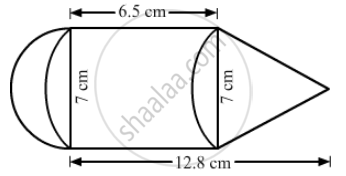Advertisement Remove all ads

# The Adjoining Figure Represents a Solid Consisting of a Cylinder Surmounted by a Cone at One End and a Hemisphere at the Other. Find the Volume of the Solid. - Mathematics

Sum

The adjoining figure represents a solid consisting of a cylinder surmounted by a cone at one end and a hemisphere at the other. Find the volume of the solid.Advertisement Remove all ads

#### Solution

Diameter of the cylindrical part =7 cm
Therefore,radius of the cylindrical part = 3.5 cm

Volume of hemisphere = 2/3pir^3 = 2/3xx22/7xx3.5xx3.5xx3.5 = 89.83  "cm"^3

Volume of the cylinder= pir^2h =22/7xx3.5xx3.5xx6.5 = 250.25  "cm"^3

Height of cone

= 12.8 - 6.5 = 6.3 cm

Volume of the cone =1/3pir^2h =1/3xx22/7xx3.5xx3.5xx6.3 = 80.85  "cm"^3

Total volume = 89.83 + 250.25 + 80.85 = 420.93  "cm"^3

Is there an error in this question or solution?
Advertisement Remove all ads

#### APPEARS IN

RS Aggarwal Secondary School Class 10 Maths
Chapter 19 Volume and Surface Area of Solids
Exercise 17A | Q 27 | Page 763
Advertisement Remove all ads
Advertisement Remove all ads
Share
Notifications

View all notifications

Forgot password?
Course